Date: Tue, 22 Apr 1997 20:11:02 -0500
Sender: Rickson

Subject: natural logarithm functions

The following two questions are some of my son's homework that he is having trouble with......any advice or assistance would be appreciated.

(eX)5=1000.............the X and 5 are exponents

lnx + ln(x+3) = ln10

Hi,

There are some basic facts that you have to know about logs and exponentials to solve these problems. First the natural logarithm and exponential functions are inverses, that is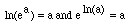.

Also there are three properties of logarithms that are very useful,

ln(ab) = ln(a) + ln(b), ln(a/b) = ln(a) - ln(b) and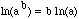.

For your first problem that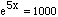take the natural logarithm of both sides to get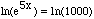, and hence 5x = ln(1000). Using the properties of logarithms this can be written in a simpler form as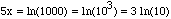so x=(3/5) ln(10).

For your son's second problem use the properties of logarithms with

ln(x) + ln(x + 3) = (10) to get ln(x(x + 3))= ln(10).

Now take the exponential of both sides and use the fact that the exponential and natural logarithm functions are inverses to arrive at x(x + 3) = 10. This expression can now be expanded to yield a quadratic equation in x that has two solutions.

You need now to take these values of x and verify that they are solutions to the original problem. The reason that this is important here is that ln(x) is only defined for positive x.

Harley

Go to Math Central

To return to the previous page use your browser's back button.